12.4. Metapopulation models

Metapopulation is a set of local populations connected by migrating individuals.

Local populations usually inhabit isolated patches of resources, and the degree of isolation may vary depending on the distance among patches: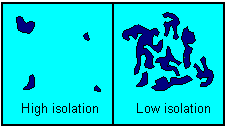Metapopulation models consider local populations as individuals. Dynamics of local populations is either not considered at all, or is considered in a very abbreviated way. Most of metapopulation models are based on colonization-extinction equilibrium.

One of the first metapopulation models was developed by MacArthur and Wilson (1967). They considered immigration of organisms (e.g., birds) from a continent to islands in the ocean. The proportion of islands colonized by a species, p, changes according to the equation: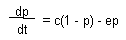Equilibrium proportion of colonized islands can be found by solving the equation dp/dt = 0: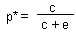Assume that extinction rate declines with increasing island diameter S:and colonization rate declines with increasing distance D from the continent: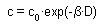Now, the proportion of colonized islands becomes a function of island size and its distance from the continent: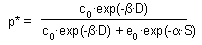If there is a group of species with similar biology and similar migration capabilities, then the proportion of colonized islands is proportional to the number of species that live on an island. Now the model can be tested using regression:where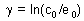Example: There are 100 bird species on the continent and the number of species on islands is in the following table:

 Island No. Diameter, km (S) Distance from continent, km (D) Number of bird species Proportion of species (p*) ln(p*/(1-p*)) 1 10 30 40 0.40 -0.405 2 3 50 5 0.05 -2.944 3 20 100 20 0.20 -1.386

Using linear regression of ln(p*/(1-p*) against two factors: S and D we get the following model parameters: α = 0.229; β = 0.0467; and γ = -1.29. These parameters can be used to predict the number of species on other islands using information about island size and its distance from the continent.

A similar metapopulation model describes metapopulation dynamics without a continent. In this case, neighboring islands become a source of colonization. The proportion of colonized islands changes according to differential equation: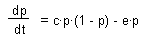where c = colonization rate per 1 island, and e = extinction rate. Equilibrium proportion of colonized islands can be found by solving the equation dp/dt = 0: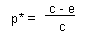This model can be expanded by incorporating island size and degree of isolation in the same way as the previous model.

Alexei Sharov 11/16/95